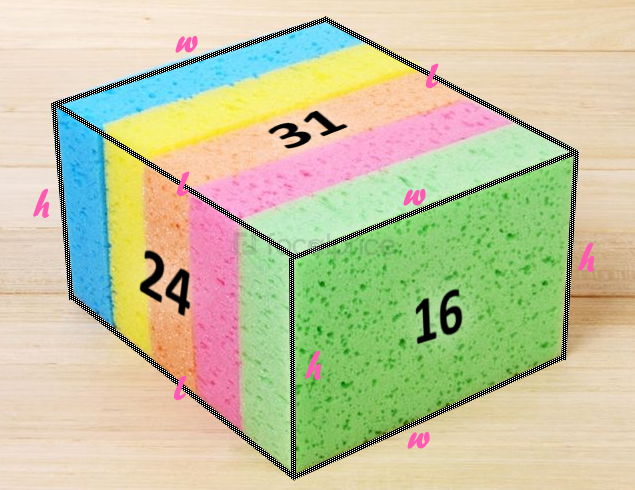# Spongy CuboidOn each face of a cuboid, the sum of its perimeter and its area is written. The numbers recorded this way are 16, 24, and 31, each written on a pair of opposite sides of the cuboid. The volume of the cuboid lies between $\text{\_\_\_\_\_\_\_\_\_\_}.$

×